# 基于 Arduino 的DIY自动喂鱼器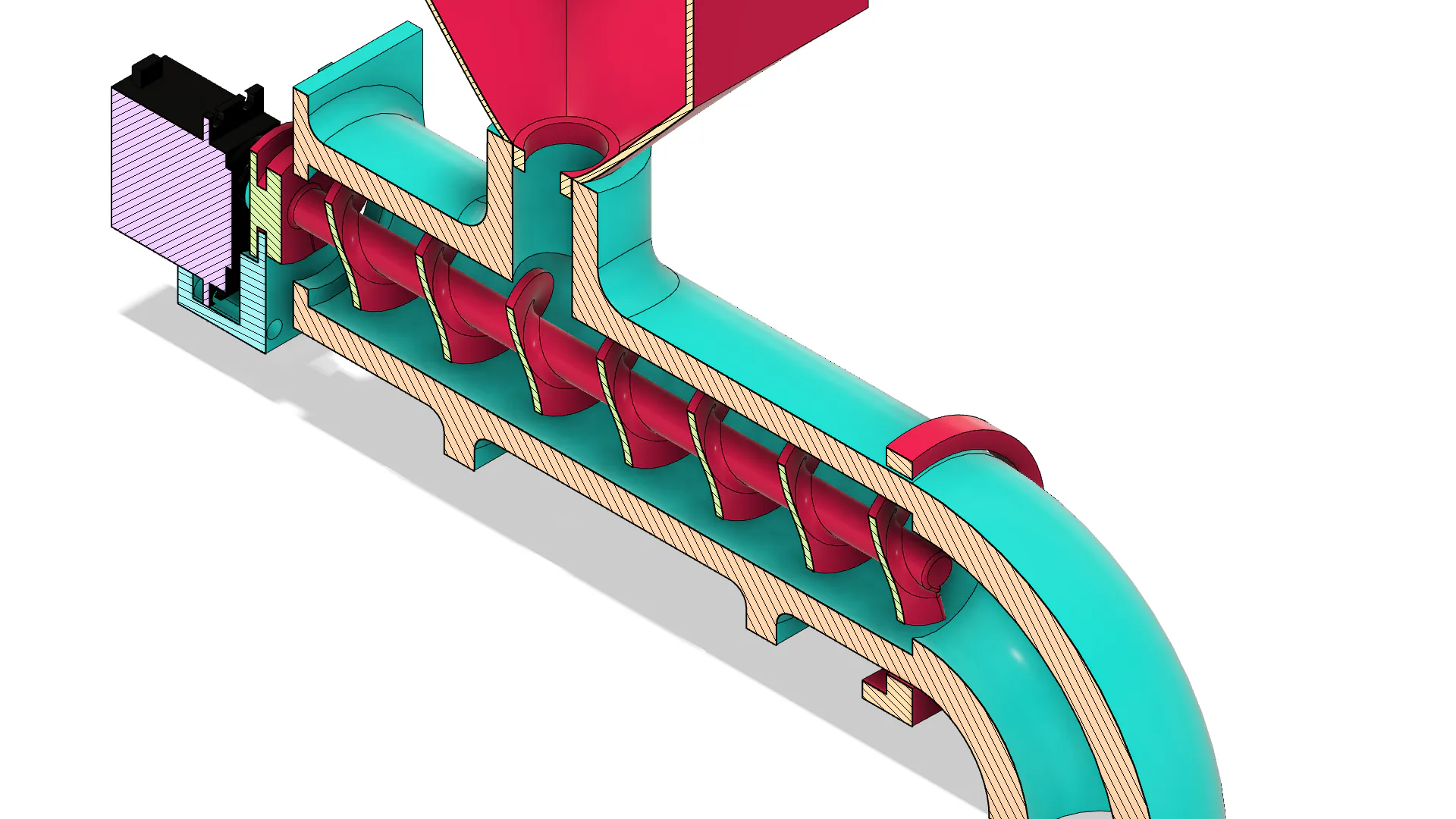Arduino DIY 喂鱼器的设计如下图所示。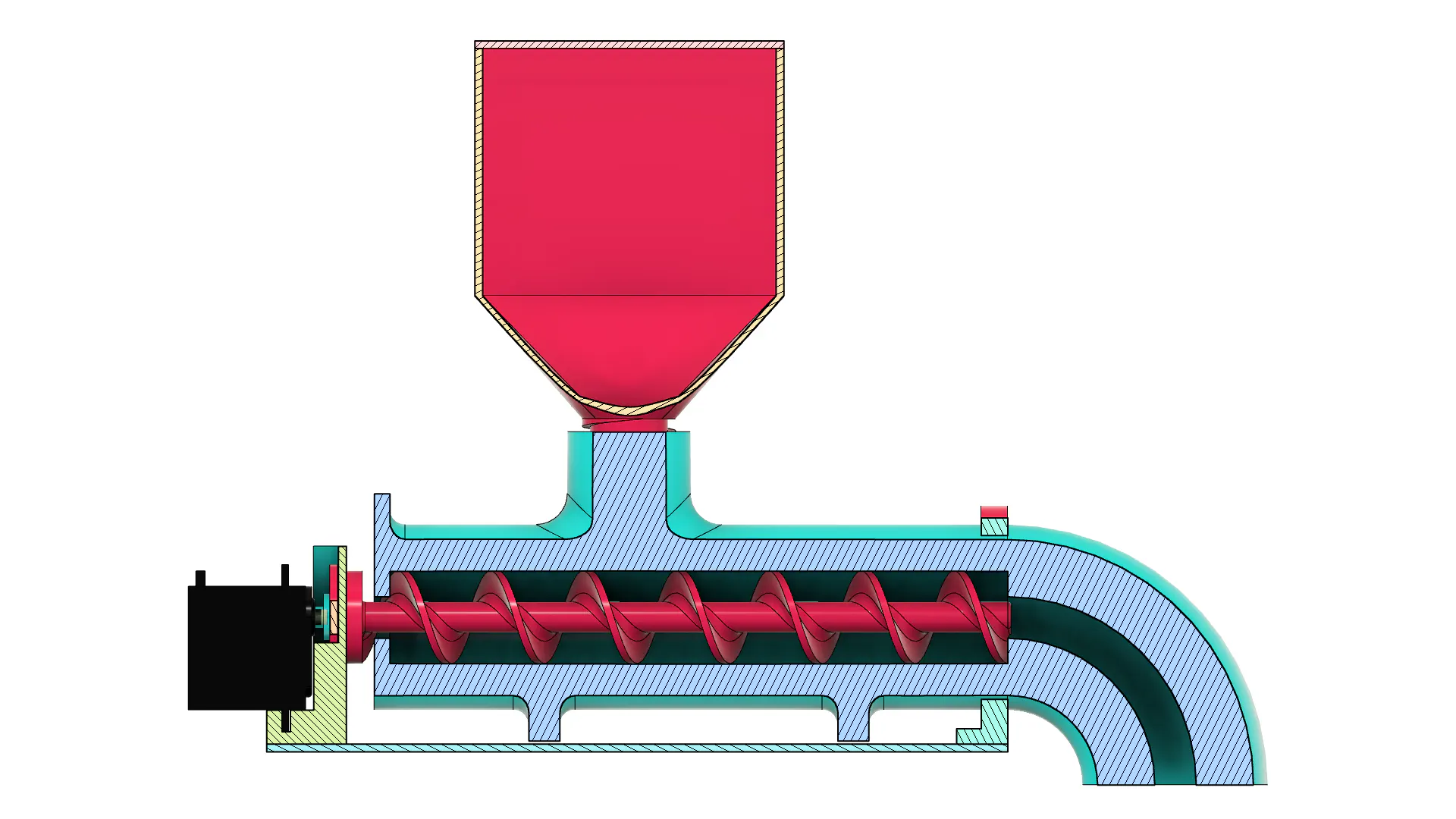Arduino DIY 喂鱼器的基础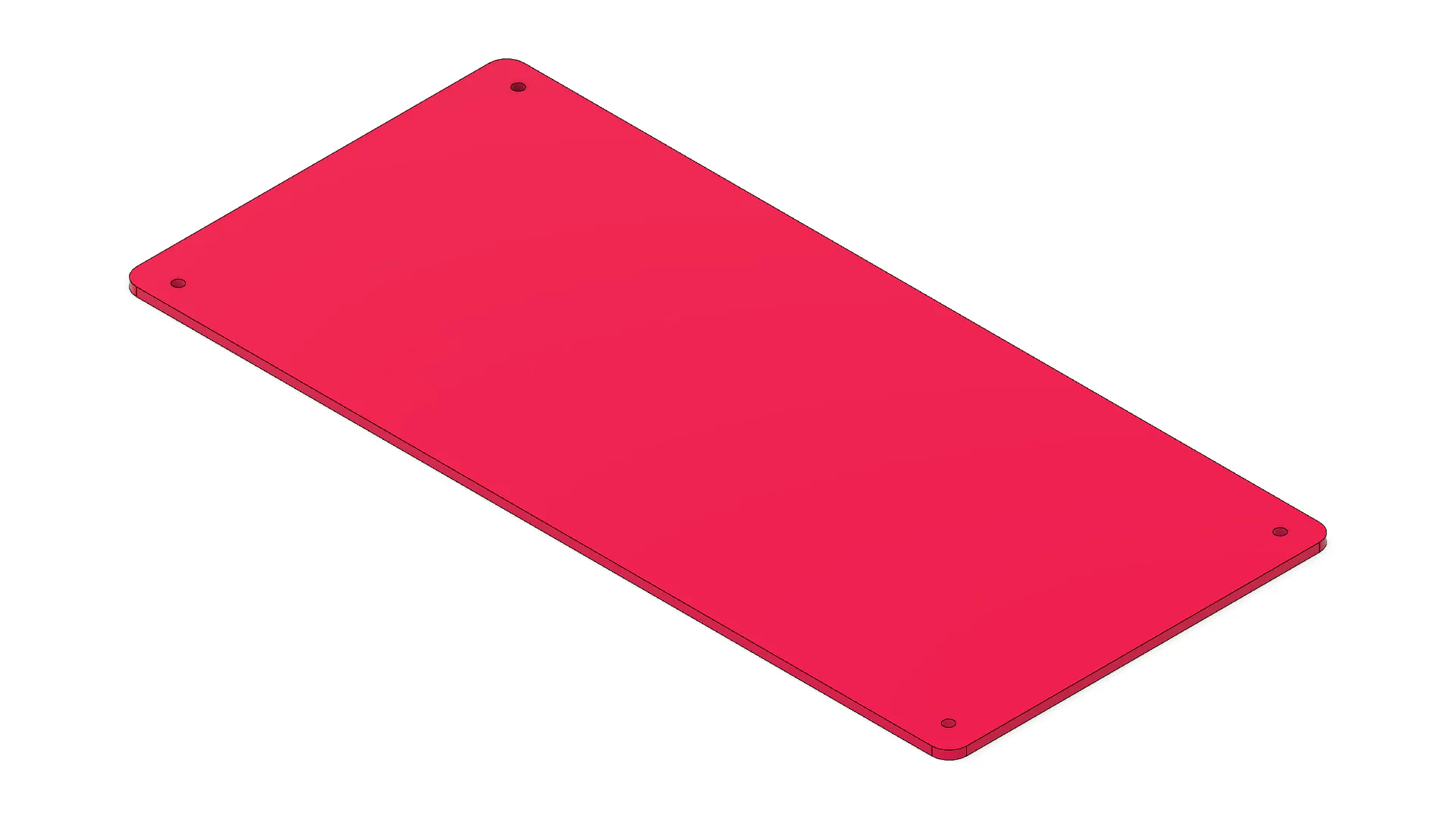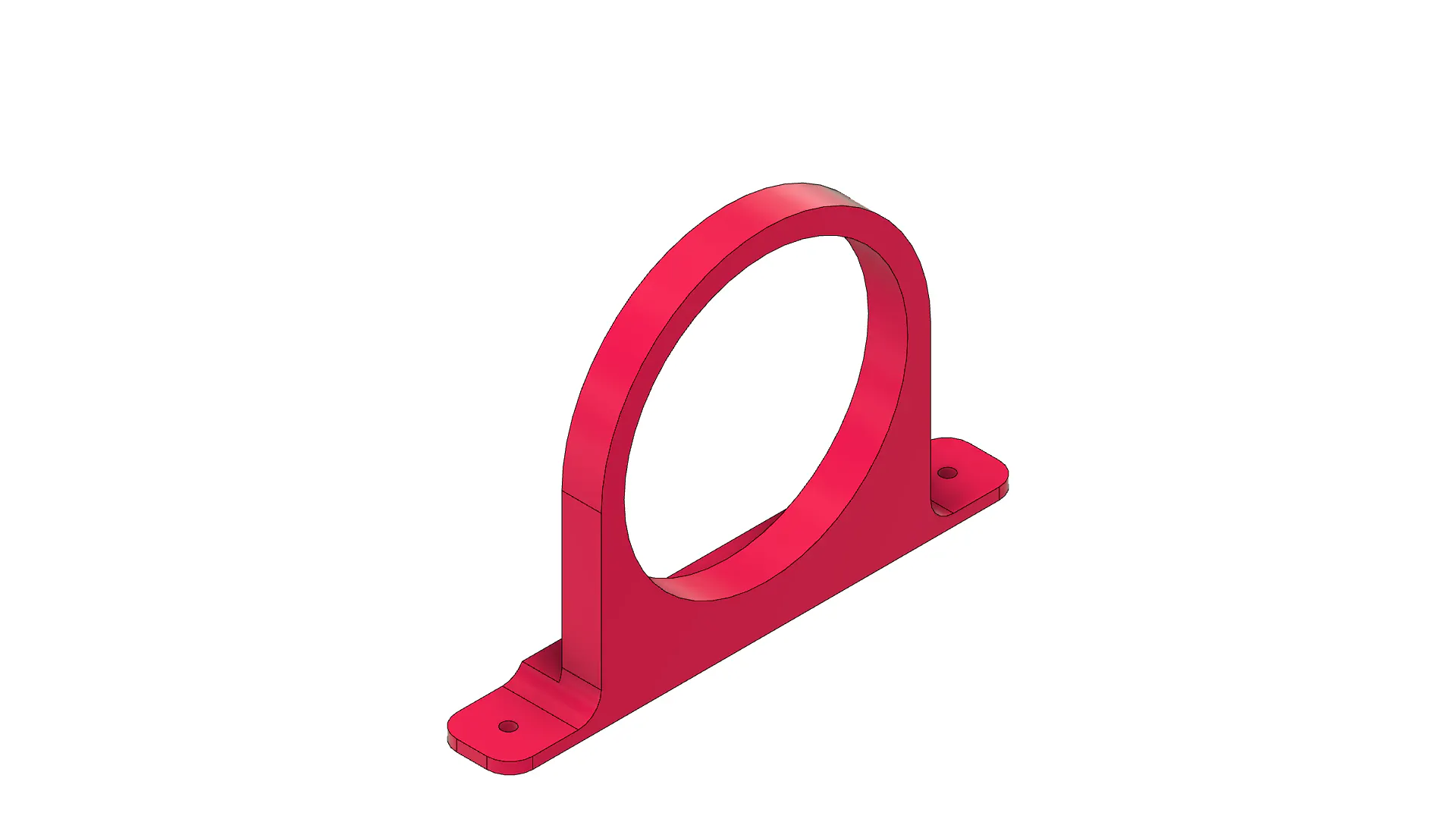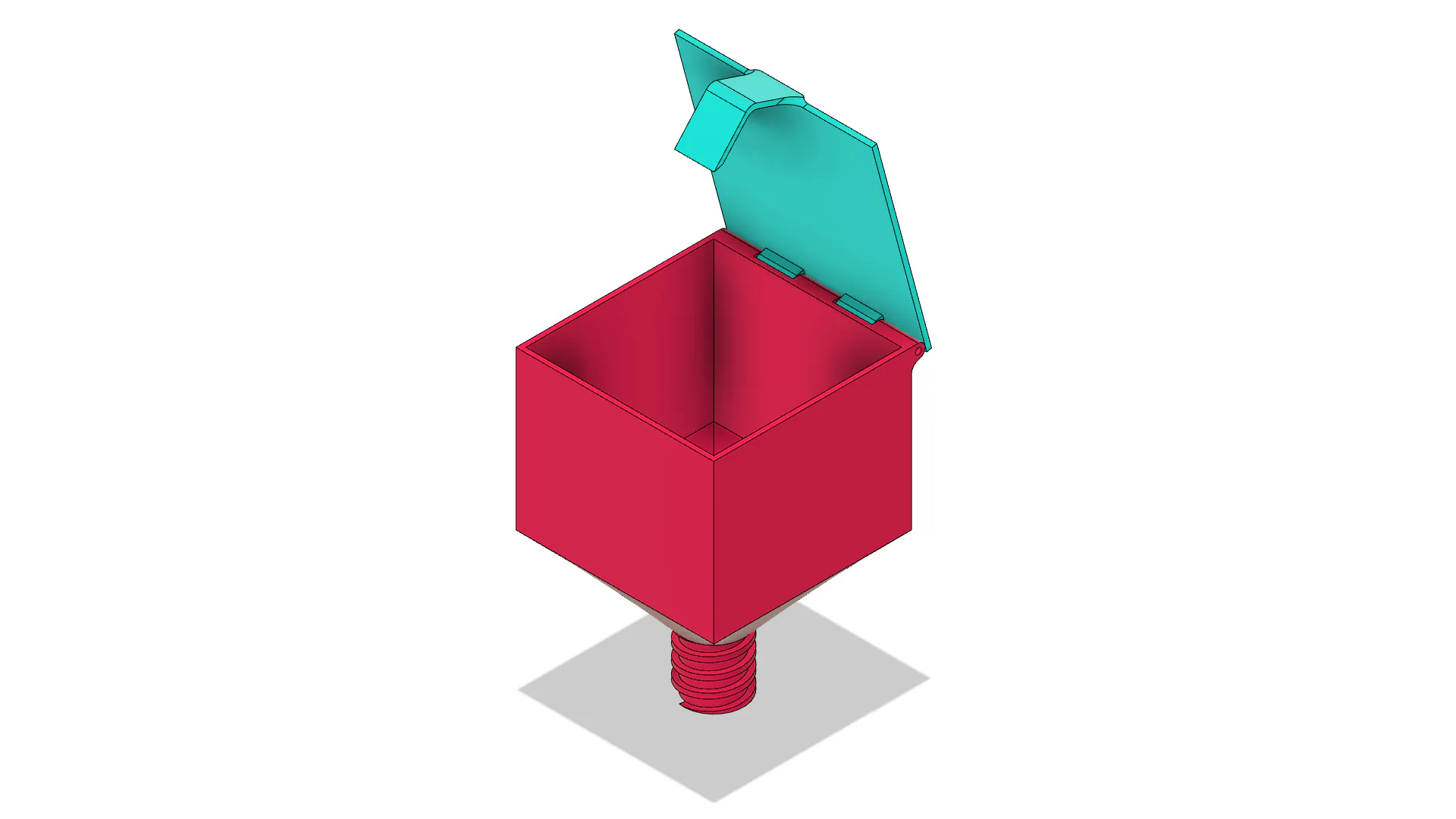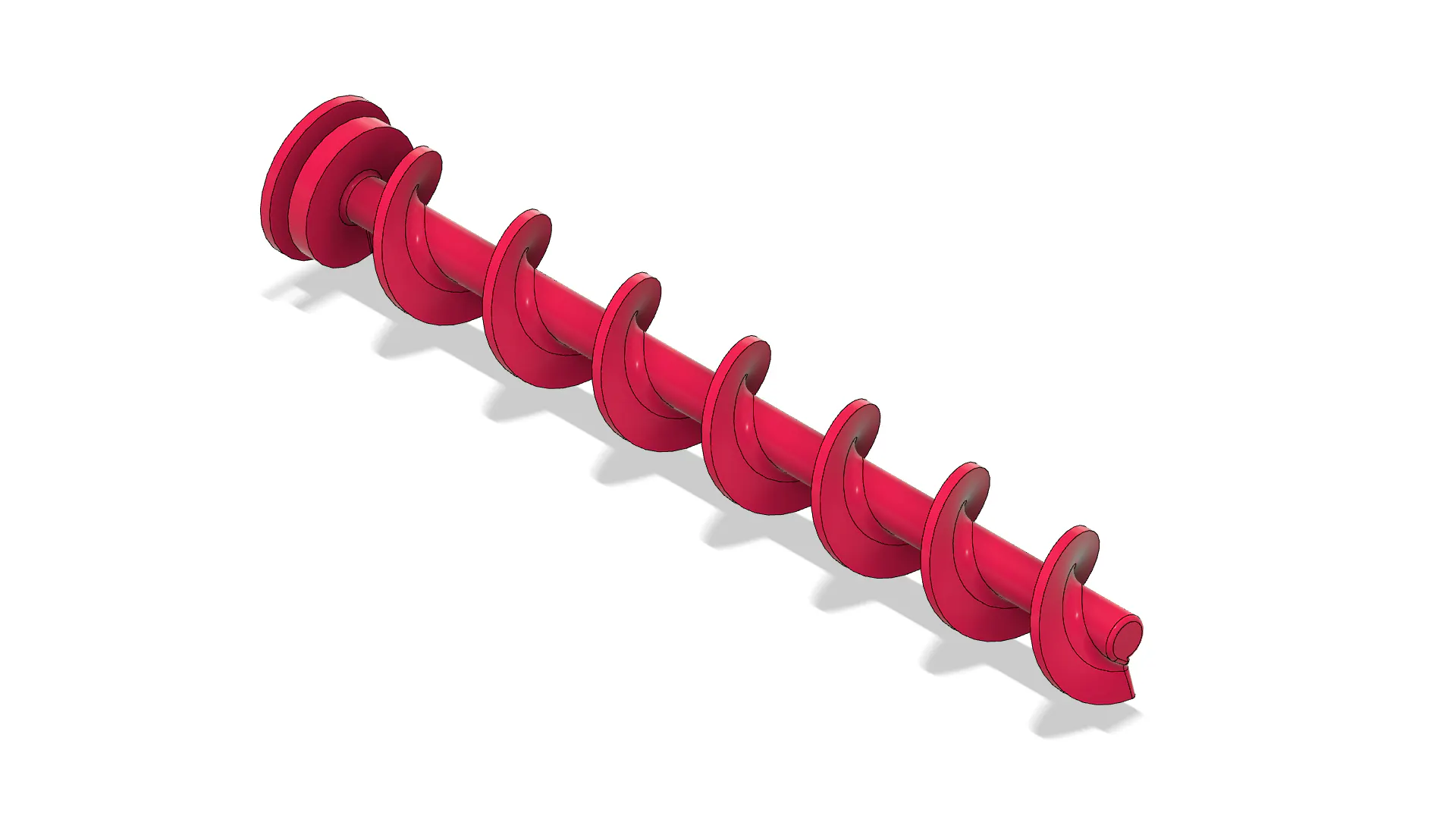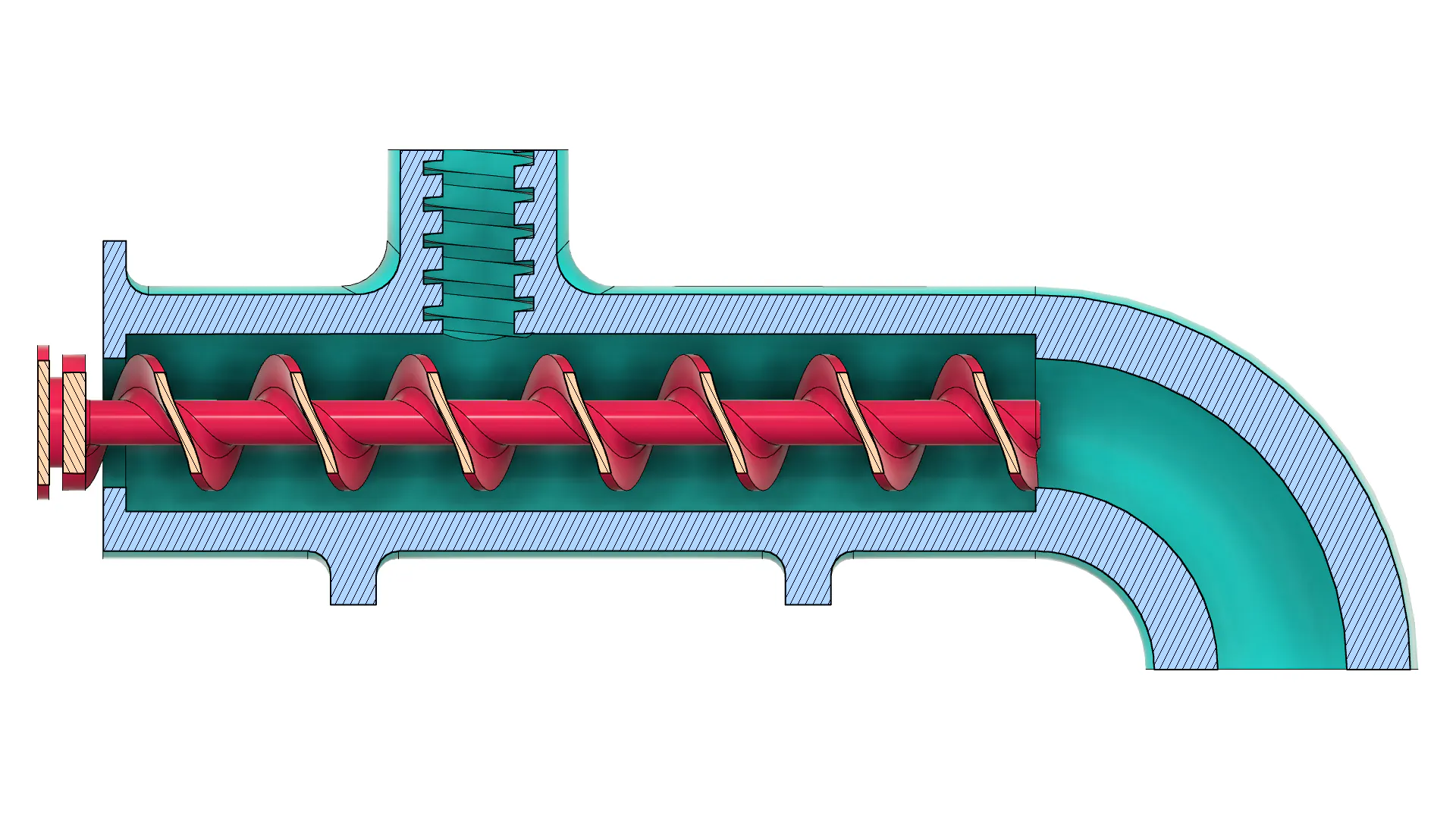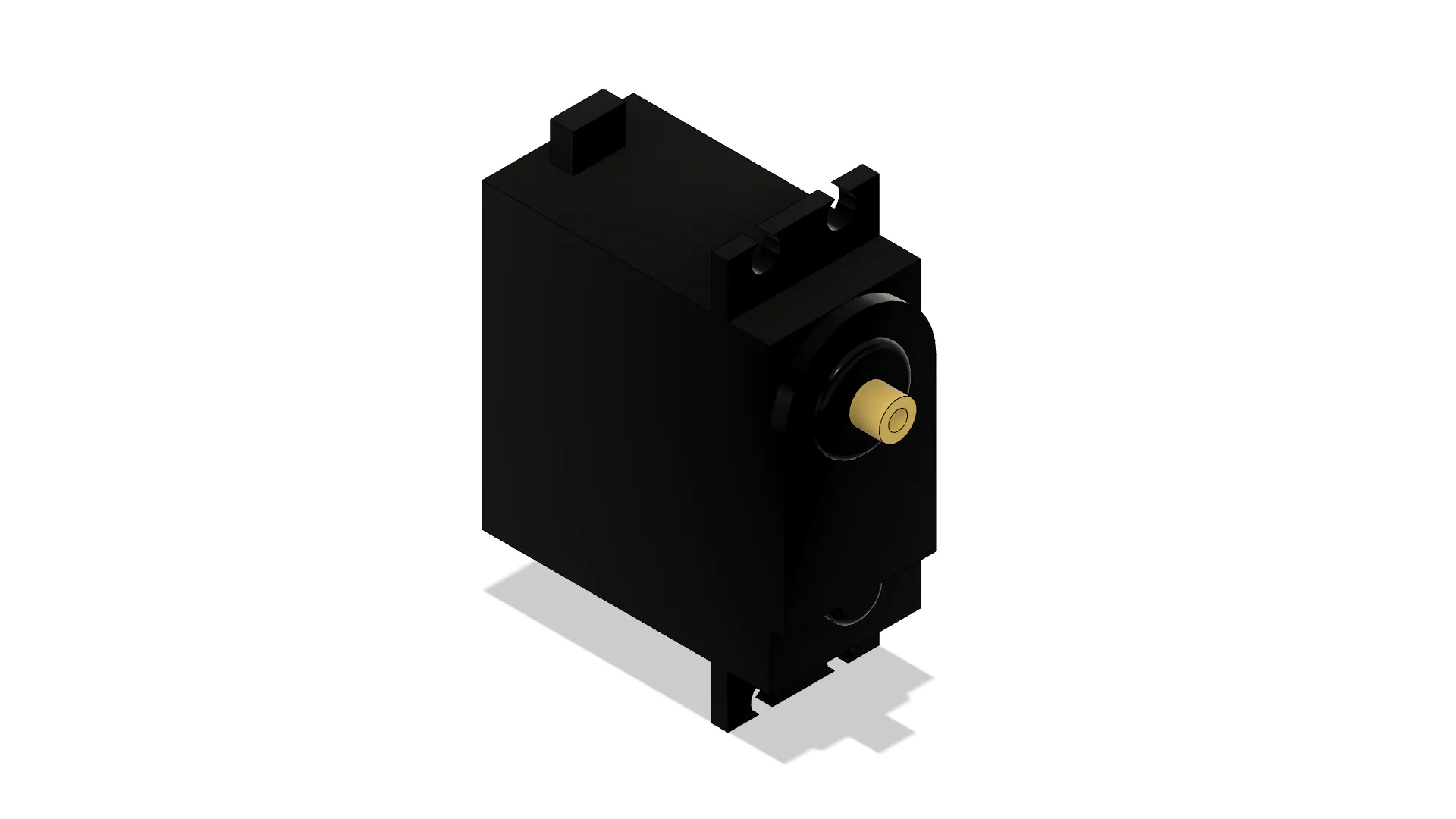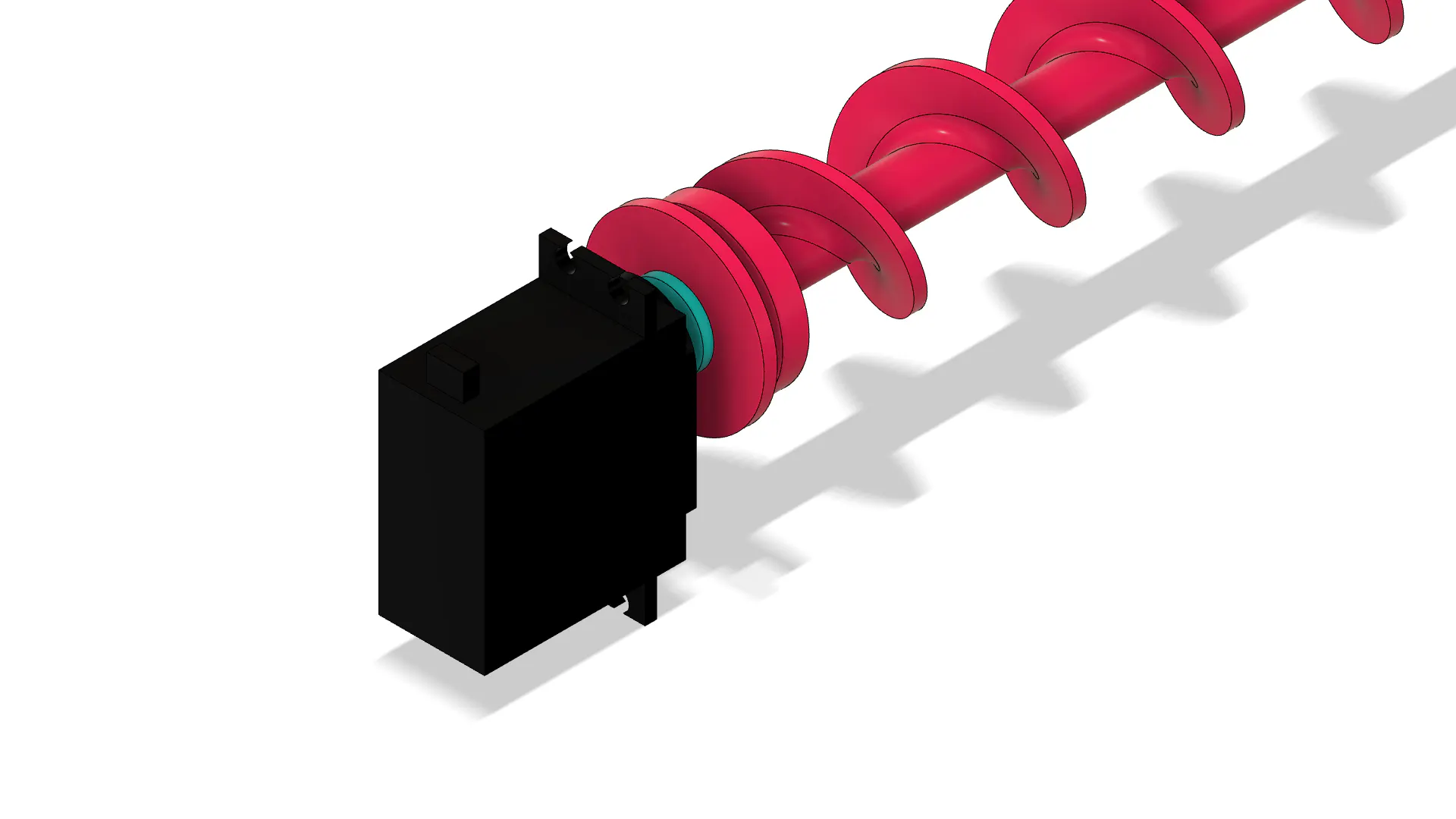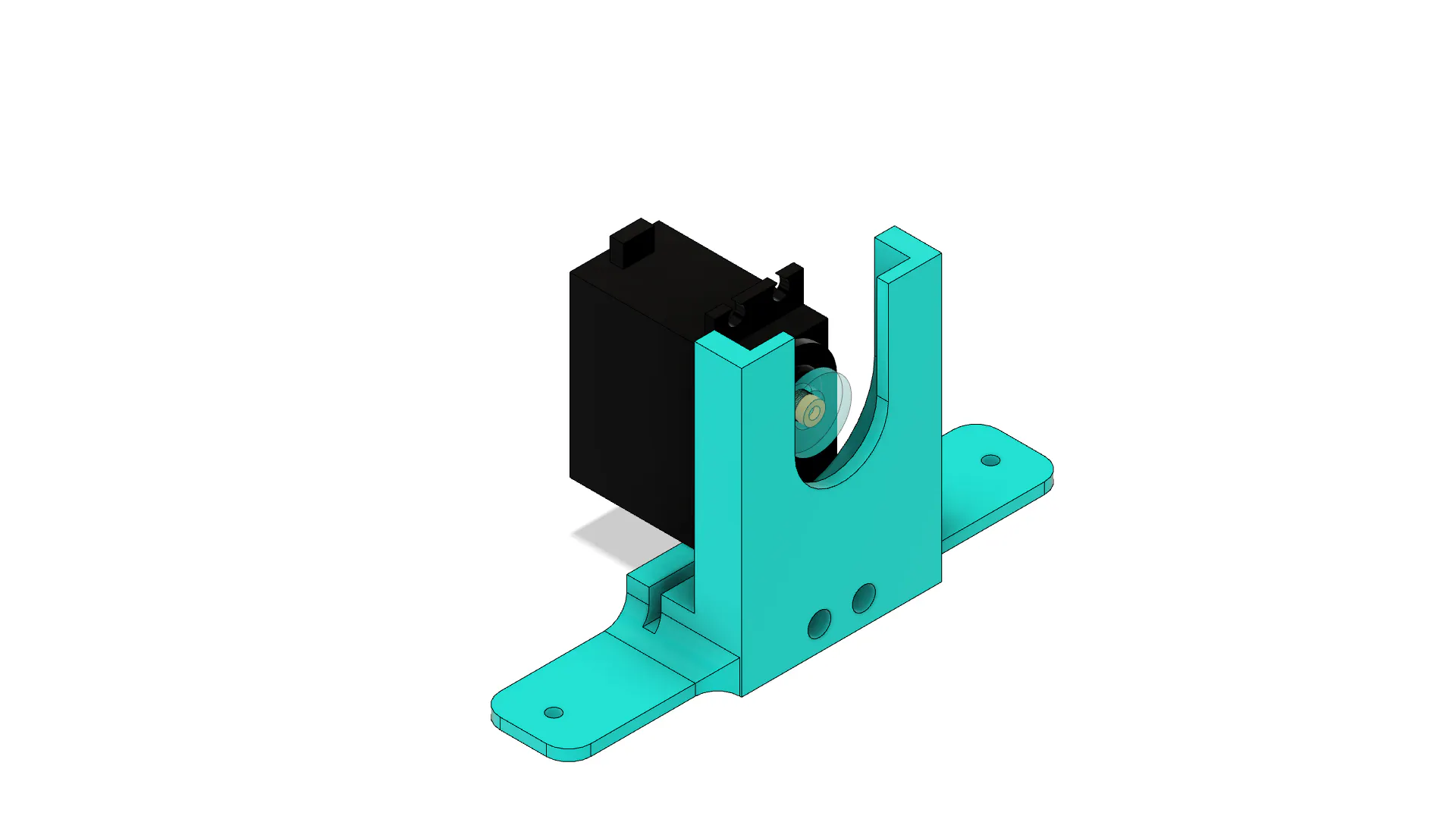#include "Servo.h"
#include <DS1307.h>
#include <Wire.h> //Biblioteca de Comunicacao I2C

int DataTime;

int actual_hour = 0, last_hour = 0;
int cont = 0;

Servo myservo;

void setup()
{

Wire.begin(); //Inicializacao da Comunicacao I2C
DS1307.begin();

DS1307.getDate(DataTime);

actual_hour = DataTime;
last_hour = DataTime;

myservo.attach(9);

}

void loop()
{

DS1307.getDate(DataTime);
actual_hour = DataTime;

if(actual_hour != last_hour)
{
cont++;
last_hour = actual_hour;
}

if(cont == 2)
{
myservo.write(180);
delay(30000);
myservo.write(90);

cont = 0;

last_hour = actual_hour;
}

}void 设置函数

void setup 函数用于设备初始化和其他设置。请参阅下面的代码部分。void循环函数中的逻辑

void 循环函数提供了使用 Arduino 控制 DIY 喂鱼器的完整逻辑。请参阅下面的代码部分。加入微信技术交流群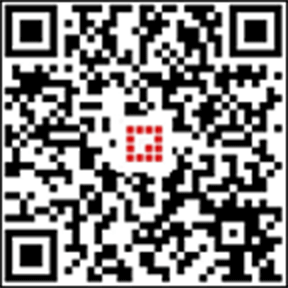关注与非网服务号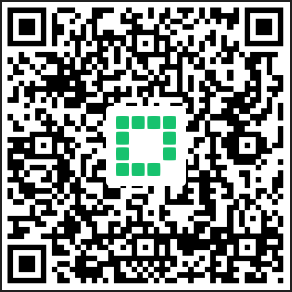加入电路城 QQ 交流群